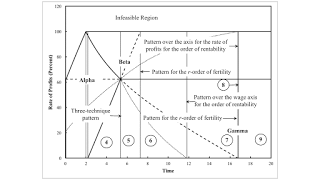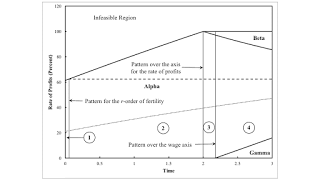Wednesday , July 28 2021Home / Post-Keynesian / Structural Dynamics With Extensive Rent

# Structural Dynamics With Extensive Rentby
Robert Vienneau
My articles My siteMy booksMy videos
Summary:
Figure 1: Variation in Switch Points with Time1.0 Introduction This post continues my effort to understand how fluke cases can partition parameter spaces in models of prices of production with extensive rent. Some background for this post is here, here, and here. 2.0 Technology The technology is described by the coefficients of production in Table 1. I assume that requirements for use are such that they cannot be satisfied by cultivating only two types of land. After fully cultivating two types, the third type must also be introduced into cultivation, at least partially. See this post for a slightly longer description of the technology. Table 1: The Coefficients of Production InputIron IndustryCorn IndustryIIIIIIIVLabora0,1 = 1a0,2 = 1/2a0,3 = 3a0,4(t) = 2.0743 e-0.03648 tType 1

Topics:
Robert Vienneau considers the following as important: ,

This could be interesting, too:

Robert Vienneau writes Extensive Rent For A Reswitching Example

Robert Vienneau writes Fluke Cases for the Order of Fertility

Robert Vienneau writes Flukes In A Modification Of An Example With Land

Robert Vienneau writes Does The Existence Of Wine Refute The Labor Theory Of Value?Figure 1: Variation in Switch Points with Time
1.0 Introduction

This post continues my effort to understand how fluke cases can partition parameter spaces in models of prices of production with extensive rent. Some background for this post is here, here, and here.

2.0 Technology

The technology is described by the coefficients of production in Table 1. I assume that requirements for use are such that they cannot be satisfied by cultivating only two types of land. After fully cultivating two types, the third type must also be introduced into cultivation, at least partially. See this post for a slightly longer description of the technology.

 Input Iron Industry Corn Industry I II III IV Labor a0,1 = 1 a0,2 = 1/2 a0,3 = 3 a0,4(t) = 2.0743 e-0.03648 t Type 1 Land 0 b1,2 = 1 0 0 Type 2 Land 0 0 b2,3 = 1 0 Type 3 Land 0 0 0 b3,4 = 1 Iron a1,1 = 0 a1,2 = 1/2 a1,3 = 1/8 a1,4(t) = 0.3551 e-0.06337 t Corn a2,1 = 1/2 a2,2 = 0 a2,3 = 0 a2,4(t) = 0.3343 e-0.2906 t

Table 2 lists the techniques for this example. Feasiblity of a technique is determined by requirements for use. Only techniques in which all three types of land must be cultivated are listed.

 Technique Type of Land Type 1 Type 2 Type 3 Alpha Fully farmed Fully farmed Partially farmed Beta Partially farmed Fully farmed Fully farmed
3.0 Prices

I consider the system of prices of production. Profits, rent and wages are paid out of the surplus at the end of the year. Each of the four processes contributes an equation to the system of price equations. A bushel corn is the numeraire, and rent must be zero on at least one type of land.

4.0 Variations of the Choice of Technique

In Figures 1 and 2, thin vertical lines partition time into numbered regions. In each numbered region, the variation of the cost-minimizing technique with distribution is qualitatively invariant. The partitions are labeled with a type of patterns of switch points. Region 8 is a narrow range of time between a pattern for the r-order of fertility, which occurs first, and a pattern over the axis for the rate of profits for the order of rentability.Figure 2: Variation in Switch Points with Time (Start Enlarged)

The maximum rate of profit, the rate of profits at switch points, and the rate of profits for which the rent per acre for the two types of land that pay rent are equal are plotted as functions of time. Heavy solid lines, aside from the bound on the infeasible region, are for switch points on the inner frontier of the wage curves. These solid lines bound areas in which the cost-minimizing technique, Alpha, Beta, or Gamma, is as shown. Dashed lines are for switch points on the outer frontier. For each technique, the dashed lines bound areas in which the order of fertility does not change. Dotted lines are for the rate of profits at which rents are equal. The dashed lines bound areas, for each technique, in which the order of rentability is invariant. Table 3 summarizes how, within each numbered region, the choice of technique, the order of fertility, and the order of rentability vary with the rate of profits.

 Region Range of r Technique Order of Fertility Order of Rentability 1 0 < r < r1,2 Alpha 1 ,2, 3 ρ1 > ρ2 > 0. ρ3 = 0 r1,2 < r < rmax, 3 ρ2 > ρ1 > 0. ρ3 = 0 2 0 < r < r1,2 Alpha 1 ,2, 3 ρ1 > ρ2 > 0. ρ3 = 0 r1,2 < r < r* ρ2 > ρ1 > 0. ρ3 = 0 r* < r < rmax, 3 2, 1, 3 3 0 < r < r1,2 Alpha 1 ,2, 3 ρ1 > ρ2 > 0. ρ3 = 0 r1,2 < r < r* ρ2 > ρ1 > 0. ρ3 = 0 r* < r < r** 2, 1, 3 r** < r < rmax, 1 Beta 2, 3, 1 ρ2 > ρ3 > 0. ρ1 = 0 4 0 < r < r* Gamma 1 ,3, 2 ρ1 > ρ3 > 0. ρ2 = 0 r* < r < r1,2 Alpha 1 ,2, 3 ρ1 > ρ2 > 0. ρ3 = 0 r1,2 < r < r** ρ2 > ρ1 > 0. ρ3 = 0 r** < r < r*** 2, 1, 3 r*** < r < rmax, 1 Beta 2, 3, 1 ρ2 > ρ3 > 0. ρ1 = 0 5 0 < r < r1,3 Gamma 1 ,3, 2 ρ1 > ρ3 > 0. ρ2 = 0 r1,3 < r < r* ρ3 > ρ1 > 0. ρ2 = 0 r* < r < r** 3, 1, 2 r** < r < r2,3 Beta 3 ,2, 1 ρ3 > ρ2 > 0. ρ1 = 0 r2,3 < r < r*** ρ2 > ρ3 > 0. ρ1 = 0 r*** < r < rmax, 1 2, 3, 1 6 0 < r < r1,3 Gamma 1 ,3, 2 ρ1 > ρ3 > 0. ρ2 = 0 r1,3 < r < r* ρ3 > ρ1 > 0. ρ2 = 0 r* < r < r** 3, 1, 2 r** < r < r2,3 Beta 3 ,2, 1 ρ3 > ρ2 > 0. ρ1 = 0 r2,3 < r < rmax,1 ρ2 > ρ3 > 0. ρ1 = 0 7 0 < r < r* Gamma 1 ,3, 2 ρ3 > ρ1 > 0. ρ2 = 0 r* < r < r** 3, 1, 2 r** < r < r2,3 Beta 3 ,2, 1 ρ3 > ρ2 > 0. ρ1 = 0 r2,3 < r < rmax,1 ρ2 > ρ3 > 0. ρ1 = 0 8 0 < r < r* Gamma 3, 1, 2 ρ3 > ρ1 > 0. ρ2 = 0 r* < r < r2,3 Beta 3 ,2, 1 ρ3 > ρ2 > 0. ρ1 = 0 r2,3 < r < rmax,1 ρ2 > ρ3 > 0. ρ1 = 0 9 0 < r < r* Gamma 3, 1, 2 ρ3 > ρ1 > 0. ρ2 = 0 r* < r < rmax,1 Beta 3 ,2, 1 ρ3 > ρ2 > 0. ρ1 = 0

Some of the fluke cases that partition the parameter space in this example arise in models with circulating capital alone and no scarce unproduced means of production. Some are specific to models with land. At the time for the partition between regions 2 and 3, a fluke switch point between the Alpha and Beta techniques occurs on the inner wage frontier with a wage of zero. This fluke is associated with the emergence of a range of low rates of profits in which the Beta technique is cost-minimizing. The partition between regions 3 and 4 is characterized by a fluke switch point on the inner wage frontier with a rate of profits of zero. This fluke is associated with the emergence of a range of high rate of profits in which the Gamma technique is cost-minimizing.

The three-technique pattern of switch points, defining the partition between regions 4 and 5, is probably the most visually noticeable fluke case depicted in Figure 8. For the coefficients of production at this instant in time, the wage curves for the Alpha, Beta, and Gamma techniques intersect at a single switch point. This switch point is simultaneously on the inner and the outer frontiers of the wage curves. For the rate of profits at which this switch point is defined, all three types of lands are equally fertile, and none of them pay any rent. None of the types of land need be fully cultivated prior to some other type being taken into cultivation.

Fluke cases I christen patterns for the r-order of fertility are specific to models of rent. A fluke switch point on the outer frontier also lies on the wage axis at the time which partitions regions 7 and 8. At this time, the range of the rate of profits in which the order of fertility is type 1, type 3, and type 2 land vanishes over the wage axis. The partition between regions 1 and 2 qualitatively resembles the partition between regions 5 and 6. A switch point on the outer frontier of wage curves occurs at a rate of profits of 100 percent, which is also the maximum rate of profits of the Beta technique. This fluke case is associated with a disappearance of a range of the rate of profits at which the order of fertility is type 2, type3, and type 1 lands.

A pattern for the w-order of fertility occurs at a time of approximately 6.0619. The switch point between type 1 and type 3 lands of the outer occurs at the same wage as the maximum wage for type 2 land. This switch point is associated with the disappearance of a range of wages at which the order of fertility is type 3, type 1, and type 2 lands, given the wage. Since the rate of profits is taken as given throughout this post, this example of a pattern for the w-order of fertility is merely an aside.

Patterns in the order of rentability are also specific to models with land. Consider the partition between regions 6 and 7. This is a pattern over the wage axis for the order of rentability. At a rate of profits of zero, the rent on an acre of type 1 and type 3 land is identical. This fluke case is associated with the disappearance of a range of the rate of profits in which the order of rentability is type 1, type 3, type 2 lands. The partition between regions 8 and 9 is a pattern over the axis for the rate of profits for the order of rentability.

5.0 Conclusions

This post continues to extend my research program. It illustrates that a divergence between the order of fertility and the order of rentability is not a fluke case.